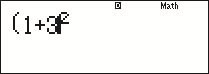Science, Maths & Technology

### Become an OU student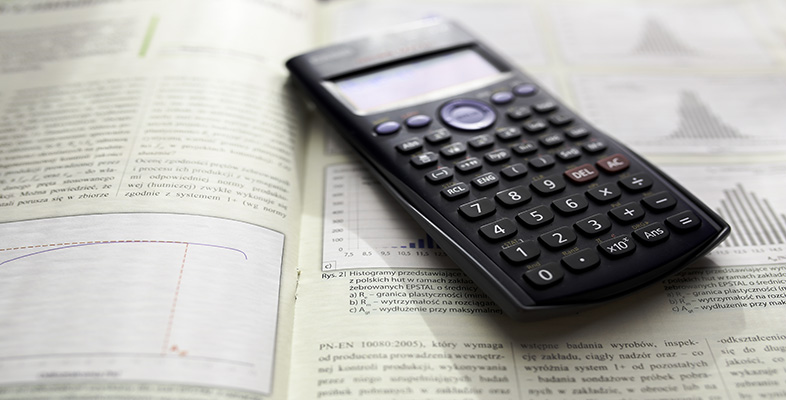Using a scientific calculator

Start this free course now. Just create an account and sign in. Enrol and complete the course for a free statement of participation or digital badge if available.

# 1.4 Making corrections

If you make a mistake when entering a key sequence into the calculator, you can use the editing facilities to correct your error.

The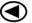and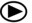keys on the large cursor control button enable you to move the cursor (shown on the display as ‘’) within a calculation on the calculator screen. Characters can then be inserted at the cursor location simply by pressing the appropriate buttons, and items to the left of the cursor can be deleted using thekey. This can be done either before or after the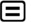key has been pressed. To re-evaluate an edited calculation, simply pressat any time.

In some circumstances, however, it may be easiest to abandon what you have typed and start again, by pressing the ‘all clear’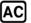key!

If a severe error is made when entering a calculation into the calculator, it may prevent the answer being calculated at all, as the calculation may not make mathematical sense. In such circumstances a ‘Syntax Error’ will be displayed as shown in Figure 6. The Syntax Error screen gives you two options:

• Pressto abandon the calculation and clear the screen
• press eitherorto return to the erroneous calculation with the editing cursor placed at the point of the error, ready for a correction to be made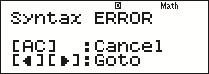Figure 6 Syntax Error

Other types of calculator error that you may encounter are:

• ‘Math Error’, when the calculation you entered makes mathematical sense but the result cannot be calculated, such as attempting to divide by zero, or when the result is too large for the calculator to handle.
• ‘Stack Error’, when your calculation is too complex to be handled in one go – in such circumstances, try to break the calculation into a number of simpler ones.

Section 4 considers how you might do this.

In these cases, the calculator will display a screen similar to that for the Syntax Error, allowing you to either abandon or correct your calculation.

## Activity 5 Making corrections

Enter the following key sequence into your calculator in an (erroneous) attempt to calculate: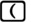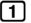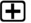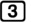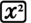What should the correct answer be, and why does this key sequence not give it?

Use the calculator editing functions to correct the inputted key sequence.

The correct value ofis 16. The key sequence given did not close the bracket before squaring the expression, so only the 3 was squared, giving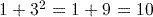.